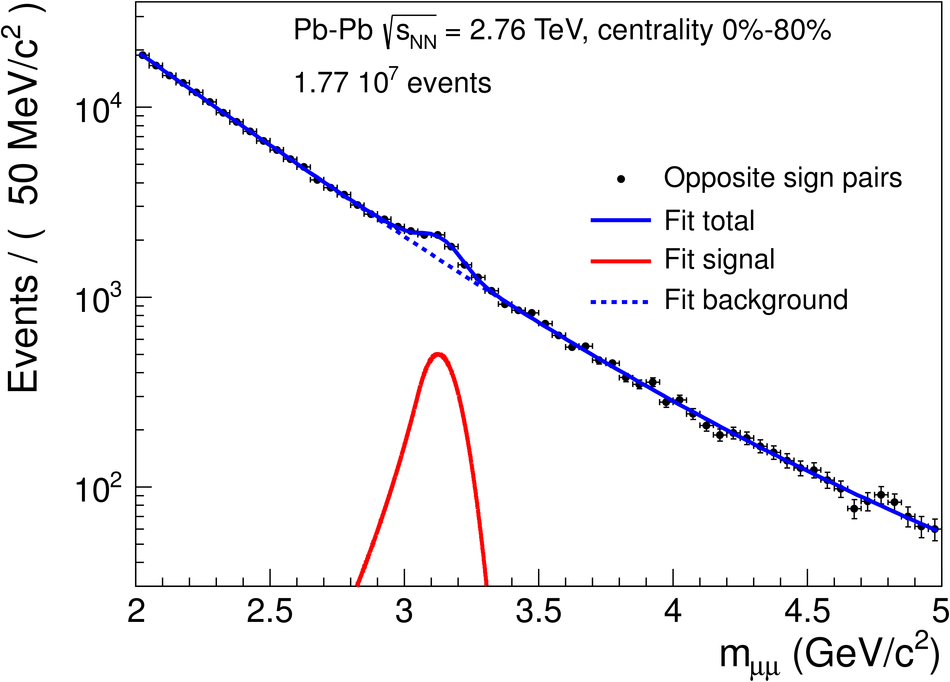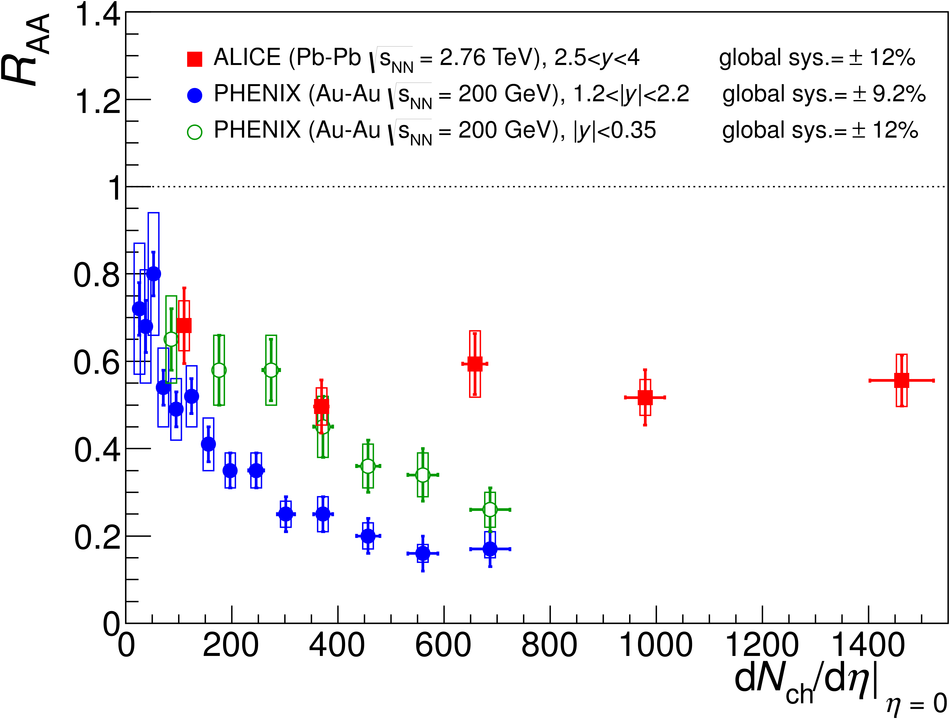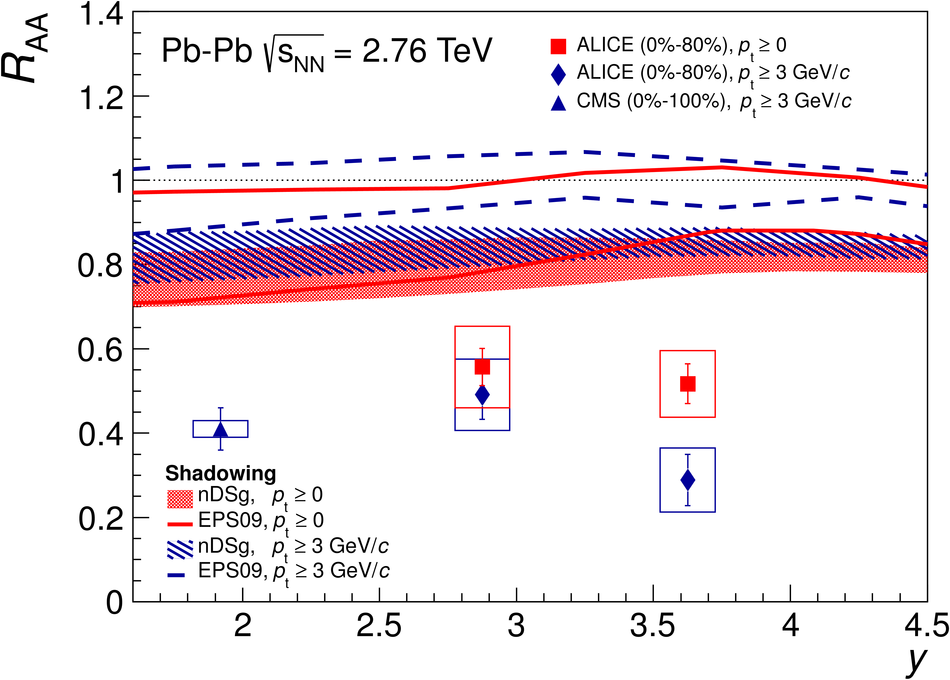# J/$ψ$ suppression at forward rapidity in Pb-Pb collisions at $\sqrt{s_{\rm NN}}$ = 2.76 TeV

The ALICE experiment has measured the inclusive J/$\psi$ production in Pb-Pb collisions at $\sqrt{s_{\mathrm{NN}} } = 2.76$ TeV down to zero transverse momentum in the rapidity range $2.5 <~ y <~ 4$. A suppression of the inclusive J/$\psi$ yield in Pb-Pb is observed with respect to the one measured in pp collisions scaled by the number of binary nucleon-nucleon collisions. The nuclear modification factor, integrated over the 0-80% most central collisions, is $0.545 \pm 0.032 \rm{(stat.)} \pm 0.083 \rm{(syst.)}$ and does not exhibit a significant dependence on the collision centrality. These features appear significantly different from measurements at lower collision energies. Models including J/$\psi$ production from charm quarks in a deconfined partonic phase can describe our data.

Figures

## Figure 1

 Invariant mass spectrum of $\mu^{+} \mu^{-}$ pairs (solid black circles)with $p_{\rm{t}} \geq 0$ and $2.5 < y < 4$ in the 0%-80% most central Pb-Pb collisions.## Figure 2

 Inclusive J/$\psi$ $R_{\rm{AA}}$ as a function of the mid-rapidity charged-particle density (top) and the number of participating nucleons (bottom) measured in Pb-Pb collisions at $\sqrt{s_{\mathrm{NN}}} = 2.76$ TeV compared to PHENIX results in Au-Au collisions at $\sqrt{s_{\mathrm{NN}}} = 200$ GeV at mid-rapidity and forward rapidity [4, 5, 20]. The ALICE data points are placed at the ${\rm d}N_{\rm{ch}}^{\rm{w}}/{\rm d}\eta\vert_{\eta=0}$ and $\langle N_{\rm{part}}^{\rm{w}} \rangle$ values defined in Table 1.## Figure 3

 Centrality integrated inclusive J/$\psi$ $R_{\rm{AA}}$ measured in Pb-Pb collisions at $\sqrt{s_{\mathrm{NN}}} = 2.76$ TeV as a function of rapidity for two $p_{\rm{t}}$ ranges. The open boxes contain the total systematic uncertainties except the ones on the integrated luminosity in the pp reference and on the $T_{\rm{AA}}$, i.e. 5.2% (8.3%) for the ALICE (CMS ) data. The two models [22, 23] predict the $R_{\rm{AA}}$ due only to shadowing effects for nDSg (shaded areas) and EPS09 (lines) nPDF respectively.## Figure 4

 Inclusive J/$\psi$ $R_{\rm{AA}}$ measured in Pb-Pb collisions at $\sqrt{s_{\mathrm{NN}}} = 2.76$ TeV compared to the predictions by Statistical Hadronization Model , Transport Model I  and II , see text for details. The ALICE data points are placed at the $\langle N_{\rm{part}}^{\rm{w}} \rangle$ values defined in Table 1.